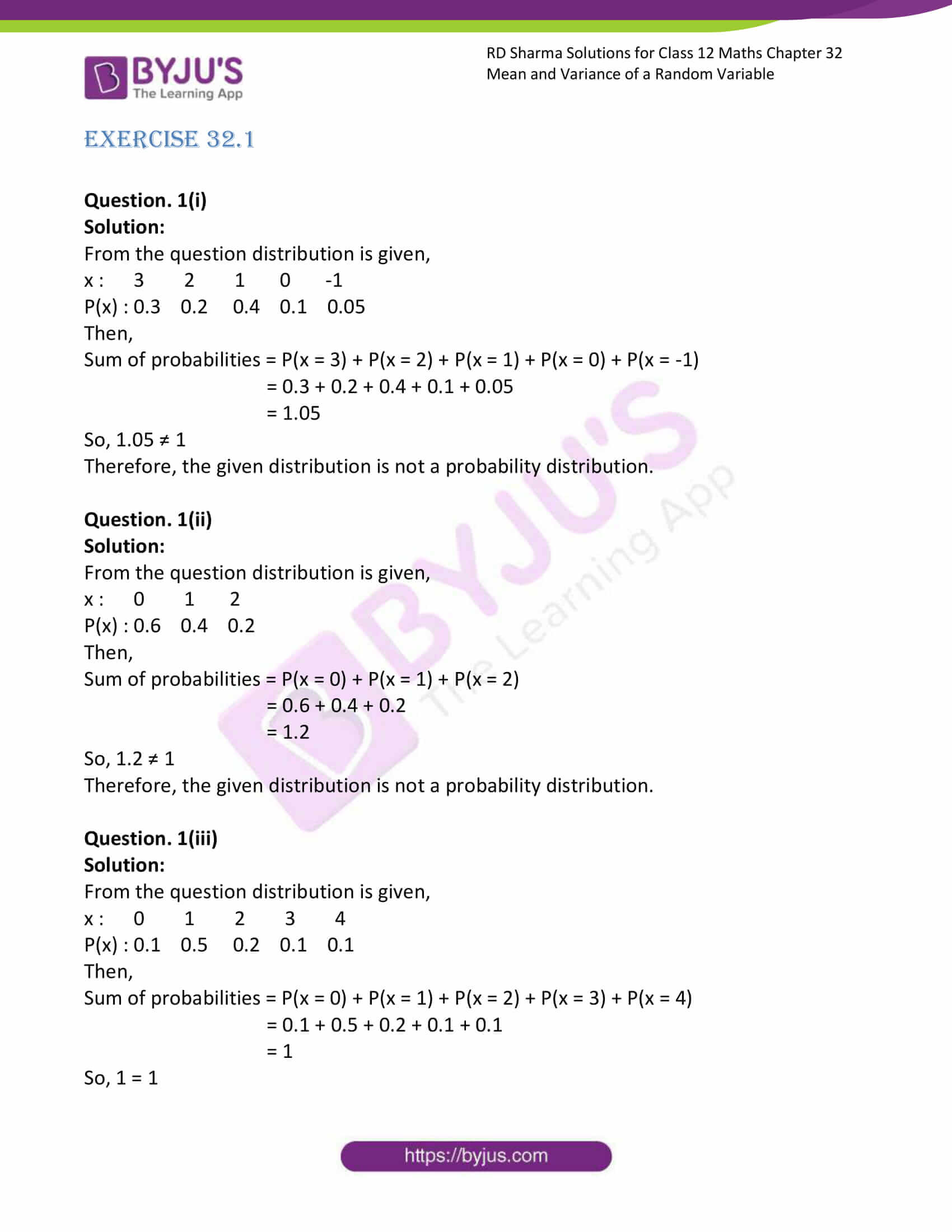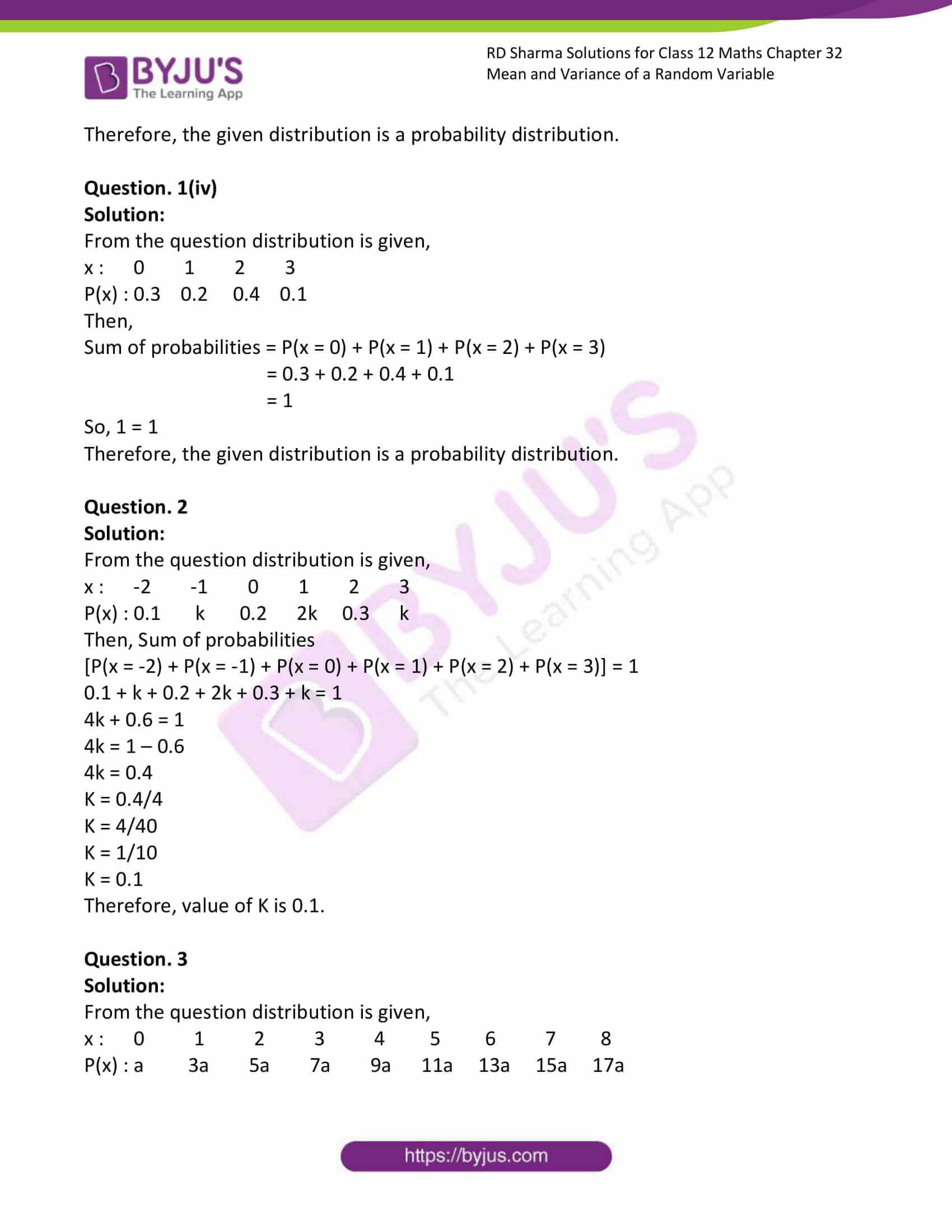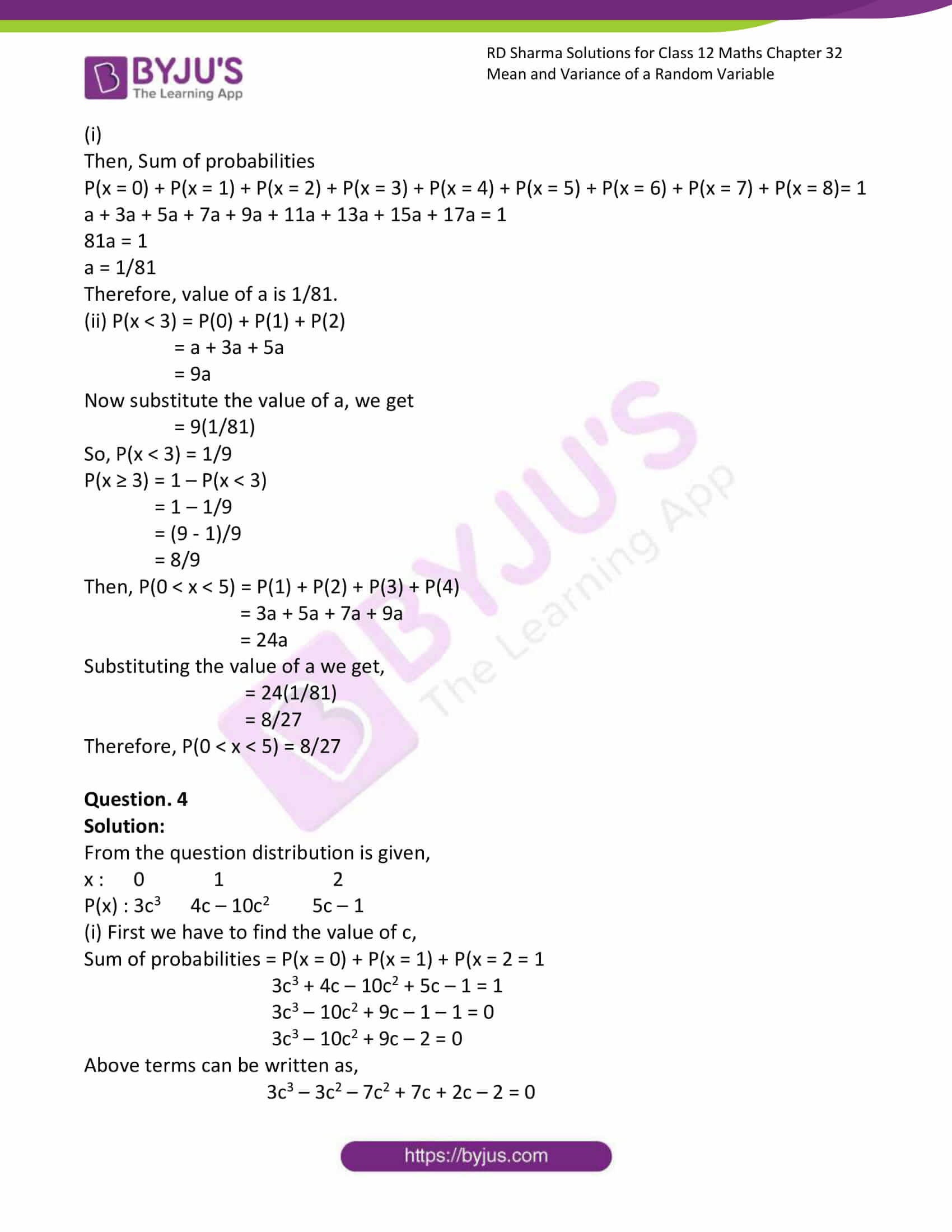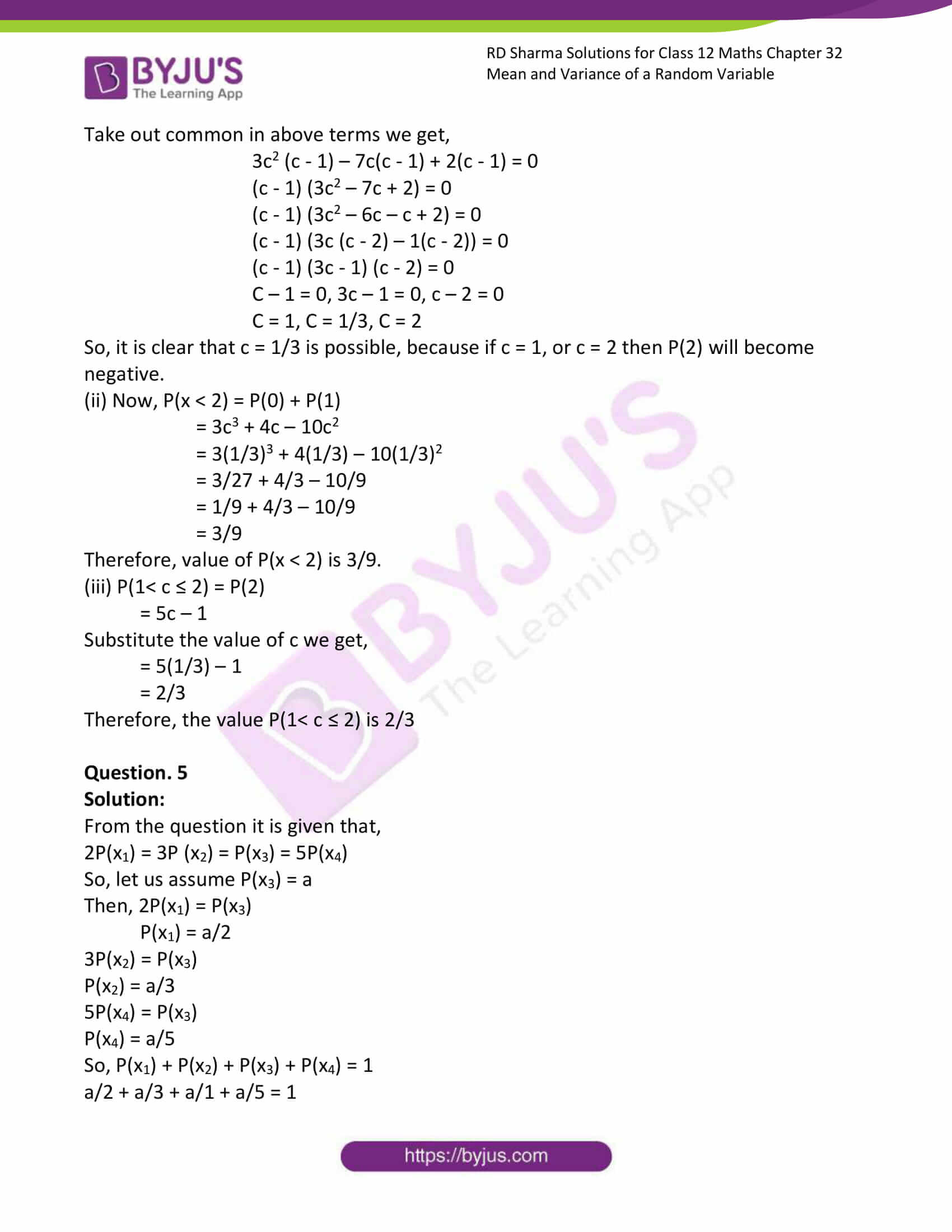# RD Sharma Solutions Class 12 Mean And Variance Of A Random Variable Exercise 32.1

RD Sharma Solutions for Class 12 Maths Exercise 32.2 Chapter 32 Mean and Variance of a Random Variable are available here. This exercise of RD Sharma Solutions for Class 12 Maths Chapter 32 contains topics related to the Mean and Standard deviation. Our expert teachers have formulated these solutions in precise, comprehensive and in a detailed form. This book is one of the top materials when it comes to providing a question bank to practice.

## Download PDF of Rd Sharma Solution for Class 12 Maths Chapter 32 Exercise 1### Access Answers for Rd Sharma Solution Class 12 Maths Chapter 32 Exercise 1

EXERCISE 32.1

Question. 1(i)

Solution:

From the question distribution is given,

x : 3 2 1 0 -1

P(x) : 0.3 0.2 0.4 0.1 0.05

Then,

Sum of probabilities = P(x = 3) + P(x = 2) + P(x = 1) + P(x = 0) + P(x = -1)

= 0.3 + 0.2 + 0.4 + 0.1 + 0.05

= 1.05

So, 1.05 ≠ 1

Therefore, the given distribution is not a probability distribution.

Question. 1(ii)

Solution:

From the question distribution is given,

x : 0 1 2

P(x) : 0.6 0.4 0.2

Then,

Sum of probabilities = P(x = 0) + P(x = 1) + P(x = 2)

= 0.6 + 0.4 + 0.2

= 1.2

So, 1.2 ≠ 1

Therefore, the given distribution is not a probability distribution.

Question. 1(iii)

Solution:

From the question distribution is given,

x : 0 1 2 3 4

P(x) : 0.1 0.5 0.2 0.1 0.1

Then,

Sum of probabilities = P(x = 0) + P(x = 1) + P(x = 2) + P(x = 3) + P(x = 4)

= 0.1 + 0.5 + 0.2 + 0.1 + 0.1

= 1

So, 1 = 1

Therefore, the given distribution is a probability distribution.

Question. 1(iv)

Solution:

From the question distribution is given,

x : 0 1 2 3

P(x) : 0.3 0.2 0.4 0.1

Then,

Sum of probabilities = P(x = 0) + P(x = 1) + P(x = 2) + P(x = 3)

= 0.3 + 0.2 + 0.4 + 0.1

= 1

So, 1 = 1

Therefore, the given distribution is a probability distribution.

Question. 2

Solution:

From the question distribution is given,

x : -2 -1 0 1 2 3

P(x) : 0.1 k 0.2 2k 0.3 k

Then, Sum of probabilities

[P(x = -2) + P(x = -1) + P(x = 0) + P(x = 1) + P(x = 2) + P(x = 3)] = 1

0.1 + k + 0.2 + 2k + 0.3 + k = 1

4k + 0.6 = 1

4k = 1 – 0.6

4k = 0.4

K = 0.4/4

K = 4/40

K = 1/10

K = 0.1

Therefore, value of K is 0.1.

Question. 3

Solution:

From the question distribution is given,

x : 0 1 2 3 4 5 6 7 8

P(x) : a 3a 5a 7a 9a 11a 13a 15a 17a

(i)

Then, Sum of probabilities

P(x = 0) + P(x = 1) + P(x = 2) + P(x = 3) + P(x = 4) + P(x = 5) + P(x = 6) + P(x = 7) + P(x = 8)= 1

a + 3a + 5a + 7a + 9a + 11a + 13a + 15a + 17a = 1

81a = 1

a = 1/81

Therefore, value of a is 1/81.

(ii) P(x < 3) = P(0) + P(1) + P(2)

= a + 3a + 5a

= 9a

Now substitute the value of a, we get

= 9(1/81)

So, P(x < 3) = 1/9

P(x ≥ 3) = 1 – P(x < 3)

= 1 – 1/9

= (9 – 1)/9

= 8/9

Then, P(0 < x < 5) = P(1) + P(2) + P(3) + P(4)

= 3a + 5a + 7a + 9a

= 24a

Substituting the value of a we get,

= 24(1/81)

= 8/27

Therefore, P(0 < x < 5) = 8/27

Question. 4

Solution:

From the question distribution is given,

x : 0 1 2

P(x) : 3c3 4c – 10c2 5c – 1

(i) First we have to find the value of c,

Sum of probabilities = P(x = 0) + P(x = 1) + P(x = 2 = 1

3c3 + 4c – 10c2 + 5c – 1 = 1

3c3 – 10c2 + 9c – 1 – 1 = 0

3c3 – 10c2 + 9c – 2 = 0

Above terms can be written as,

3c3 – 3c2 – 7c2 + 7c + 2c – 2 = 0

Take out common in above terms we get,

3c2 (c – 1) – 7c(c – 1) + 2(c – 1) = 0

(c – 1) (3c2 – 7c + 2) = 0

(c – 1) (3c2 – 6c – c + 2) = 0

(c – 1) (3c (c – 2) – 1(c – 2)) = 0

(c – 1) (3c – 1) (c – 2) = 0

C – 1 = 0, 3c – 1 = 0, c – 2 = 0

C = 1, C = 1/3, C = 2

So, it is clear that c = 1/3 is possible, because if c = 1, or c = 2 then P(2) will become negative.

(ii) Now, P(x < 2) = P(0) + P(1)

= 3c3 + 4c – 10c2

= 3(1/3)3 + 4(1/3) – 10(1/3)2

= 3/27 + 4/3 – 10/9

= 1/9 + 4/3 – 10/9

= 3/9

Therefore, value of P(x < 2) is 3/9.

(iii) P(1< c ≤ 2) = P(2)

= 5c – 1

Substitute the value of c we get,

= 5(1/3) – 1

= 2/3

Therefore, the value P(1< c ≤ 2) is 2/3

Question. 5

Solution:

From the question it is given that,

2P(x1) = 3P (x2) = P(x3) = 5P(x4)

So, let us assume P(x3) = a

Then, 2P(x1) = P(x3)

P(x1) = a/2

3P(x2) = P(x3)

P(x2) = a/3

5P(x4) = P(x3)

P(x4) = a/5

So, P(x1) + P(x2) + P(x3) + P(x4) = 1

a/2 + a/3 + a/1 + a/5 = 1

LCM of 2, 3, 1 and 5 is 30.

(15a + 10a + 30a + 6a)/30 = 1

61a = 30

a = 30/61

Therefore,

x : x1 x2 x3 x4

P(x) : 15/61 10/61 30/61 6/61